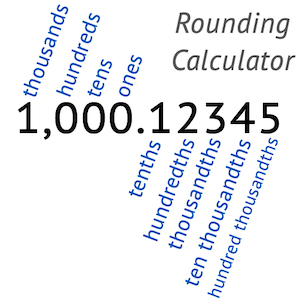# Rounding Calculator

Enter the number and select the rounding digits to calculate the approximate rounding value.

0.0## How to Calculate Rounding?

• Find the digit you want to round.
• Check the number before the rounding digit.
• If the previous number is less than 5 (1,2,3,4) no round needed.
• If the previous number is greater than 4 (5,6,7,8,9) round up and add 1.

### Rounding to the Nearest Tenth

The tenth number is the first digit after decimal point. If the second digit is greater than or equal to 5 add 1 to calculate rounding to nearest tenth.

Example: 10,27
Second digit is 7, add 1 to 2, we get 10,3.

### Rounding to the Nearest Hundredth

The hundredth number is the second digit after decimal point. If the third digit is greater than or equal to 5 add 1 to calculate rounding to nearest hundredth.

Example: 10,276
Third digit is 6, add 1 to 7, we get 10,28.

### Rounding to the Nearest Thousandth

The thousandth number is the third digit after decimal point. If the fourth digit is greater than or equal to 5 add 1 to calculate rounding to nearest thousandth.

Example: 10,2762
Forth digit is 2, no need to add 1, we get 10,276.

### Rounding to the Nearest Ones Whole Number

The ones number is the first digit before the decimal point. If the first digit after decimal point is greater than or equal to 5 add 1 to tens digit to calculate rounding to nearest ones number.

Example: 10,7
First digit after decimal point is 7, add 1 to 0 and we get 11.

### Rounding to the Nearest Tens Whole Number

The tens number is the second digit before the decimal point. If the first digit before decimal point is greater than or equal to 5, add 1 to tens digit to calculate rounding to nearest tens number.

Example: 1067
Ones digit of the number is 7, add 1 tens digit and we get 1070.